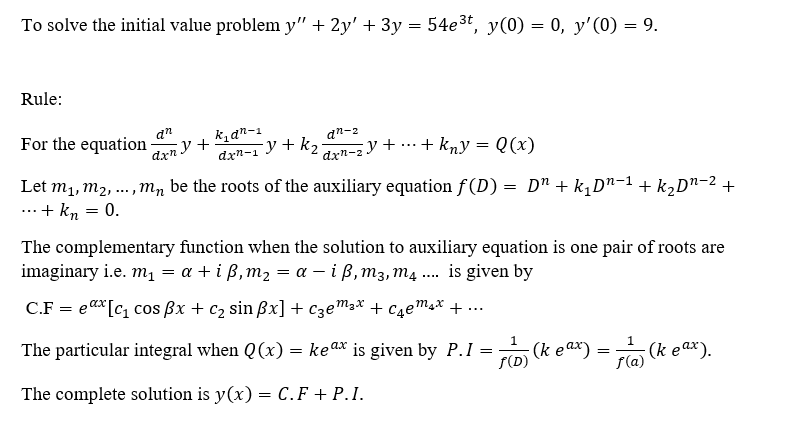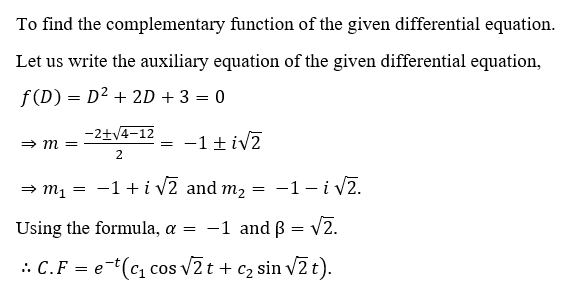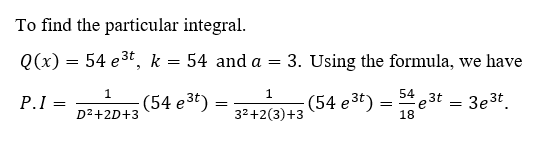# Solve the initial value problem. Describe the behavior as t approaches positive infinity.y''+2y'+3y = 54e^(3t)          y(0)=0, y'(0)=9

Question
1 views

Solve the initial value problem. Describe the behavior as t approaches positive infinity.

y''+2y'+3y = 54e^(3t)          y(0)=0, y'(0)=9

check_circle

star
star
star
star
star
1 Rating
Step 1Step 2Step 3...

### Want to see the full answer?

See Solution

#### Want to see this answer and more?

Solutions are written by subject experts who are available 24/7. Questions are typically answered within 1 hour.*

See Solution
*Response times may vary by subject and question.
Tagged in